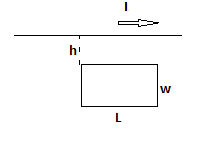# a. Determine the magnetic flux through the rectangular loop of width w and length L due to...

## Question:

a. Determine the magnetic flux through the rectangular loop of width {eq}w {/eq} and length {eq}L {/eq} due to current {eq}I {/eq} in the wire.

b. Suppose the current in wire is changing with time according to {eq}I = a + bt, {/eq} where {eq}a {/eq} and {eq}b {/eq} are constants. Determine the magnitude of emf induced in the loop for {eq}a = 2 \space A, b = -9 \space A/s, h = 1.00 \space cm, w = 10 \space cm {/eq} and {eq}L = 1.00 \space m. {/eq}

c. What is the direction of induced current in the rectangle?## Magnetic Flux

The magnetic flux is defined as the number of magnetic field lines linked with a closed surface area. Mathematically the magnetic field lines linked with an area is given by

{eq}\begin{align} \Phi_B = \int \vec{B}.d \vec{A} \end{align} {/eq}

Where B is the magnetic field and A is the area through which the flux has to be calculated.

Data Given

• Current in the wire {eq}I = a + bt {/eq}
• Value of constant a {eq}a = 2 \ \rm A {/eq}
• value of constant b {eq}b = -9 \ \rm A/s {/eq}
• Value of distance {eq}h = 1.00 \ \rm cm = 0.01 \ \rm m {/eq}
• Width of the loop {eq}W = 10 \ \rm cm = 0.10 \ \rm m {/eq}
• Length of the loop {eq}L = 1.0 \ \rm m {/eq}

Part A) To find the magnetic flux at the position of the loop due to a long straight current-carrying wire is given by using Biot-Savart Law

{eq}\begin{align} B = \frac{\mu_0 I}{2 \pi r} \end{align} {/eq}

Now the flux linked with the loop ( as we know that the magnetic field changes as we move along the width of the loop )

{eq}\begin{align} \Phi_B = \int B.dA \\ \Phi_B = \int B.L.dr \\ \Phi_B = \int_{h}^{h+W} \frac{\mu_0 IL}{2\pi} \frac{dr}{r} \\ \Phi_B = \frac{\mu_0IL}{2\pi} \left ( \ln r \right )_{h}^{h+W} \\ \color{blue}{\boxed{ \Phi_B = \frac{\mu_0 IL}{2 \pi} ln \left ( \frac{h +W}{W} \right ) }} \end{align} {/eq}

Part B) Using the Faraday's Second law of electromagnetic induction

{eq}\begin{align} \epsilon = \frac{d\Phi_B}{dt} \\ \epsilon = \frac{d}{dt}\frac{\mu_0 IL}{2 \pi} ln \left ( \frac{h +W}{W} \right ) \\ \epsilon =\frac{\mu_0 L}{2 \pi} ln \left ( \frac{h +W}{W} \right ) \frac{d (a + bt)}{dt} \\ \epsilon =\frac{\mu_0 Lb}{2 \pi} ln \left ( \frac{h +W}{W} \right ) \\ \epsilon =\frac{4\pi \times 10^{-7} \ \rm TmA^{-1} \times 1 \ \rm m \times (-9 A/s)}{2 \pi} ln \left ( \frac{0.01\ \rm m +0.10 \ \rm m}{0.10 \ \rm m} \right ) \\ \epsilon = 1.72 \times 10^{-7} \ \rm V \end{align} {/eq}

Part C) Using the Lez's law and right-hand thumb rule, the direction of the induced current in the loop will be counterclockwise.